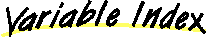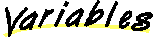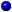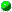`All Packages  Class Hierarchy  This Package  Previous  Next  Index  `

# Class Supporting.Numerical

```java.lang.Object
|
+----Supporting.Numerical
```

public final class Numerical
extends Object
Class that contains a selection of numerical routines.

##TRIALS
The number of witnesses queried in randomized primality test.

##Numerical()

##gcd(long, long)
Return the greatest common divisor.inverse(long, long)
Solve ax == 1 (mod n), assuming gcd( a, n ) = 1.isPrime(long)
Randomized primality test.power(long, long, long)
Return x^n (mod p) Assumes x, n >= 0, p > 0, x < p, 0^0 = 1 Overflow may occur if p > 31 bits.

##TRIALS
`public static final int TRIALS`
The number of witnesses queried in randomized primality test.

##Numerical
`public Numerical()`

##power
```public static long power(long x,
long n,
long p)```
Return x^n (mod p) Assumes x, n >= 0, p > 0, x < p, 0^0 = 1 Overflow may occur if p > 31 bits.isPrime
`public static boolean isPrime(long n)`
Randomized primality test. Adjust TRIALS to increase confidence level.

Parameters:
n - the number to test.
Returns:
if false, n is definitely not prime. If true, n is probably prime.gcd
```public static long gcd(long a,
long b)```
Return the greatest common divisor.inverse
```public static long inverse(long a,
long n)```
Solve ax == 1 (mod n), assuming gcd( a, n ) = 1.

Returns:
x.

`All Packages  Class Hierarchy  This Package  Previous  Next  Index  `